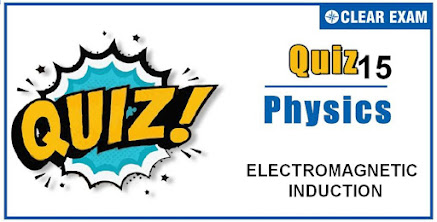## [LATEST]\$type=sticky\$show=home\$rm=0\$va=0\$count=4\$va=0

As per analysis for previous years, it has been observed that students preparing for NEET find Physics out of all the sections to be complex to handle and the majority of them are not able to comprehend the reason behind it. This problem arises especially because these aspirants appearing for the examination are more inclined to have a keen interest in Biology due to their medical background. Furthermore, sections such as Physics are dominantly based on theories, laws, numerical in comparison to a section of Biology which is more of fact-based, life sciences, and includes substantial explanations. By using the table given below, you easily and directly access to the topics and respective links of MCQs. Moreover, to make learning smooth and efficient, all the questions come with their supportive solutions to make utilization of time even more productive. Students will be covered for all their studies as the topics are available from basics to even the most advanced. .

Q1. Faraday’s laws are consequence of conservation of
•  Energy
•  Energy and magnetic field
•  Charge
•  Magnetic field
Solution
Faraday’s laws involve conversion of mechanical energy into electrical energy. This is in accordance with the law of conservation of energy

Q2. Turn ratio is 1.25. The step up transformer operates at 230 V and current through secondary is 2 A. Then current in primary is
•  25 A
•  100 A
•  50 A
•  20 A
Q3.  A copper rod of length l is rotated about one end perpendicular to the magnetic field B with constant angular velocity ω. The induced e.m.f. between the two ends is
•  1/2 Bωl2
•  3/4 Bωl2
•  Bωl2
•  2Bωl2
Solution
If in time t, the rod turns by an angle θ, the area generated by the rotation of rod will be =1/2 l×lθ=1/2 l2θ
So the flux linked with the area generated by the rotation of rod ϕ=B(1/2 l2θ) cos⁡0=1/2 Bl2θ=1/2 Bl2ωt And so e=dϕ/dt=d/dt (1/2 Bl2ωt)=1/2 Bl2ω

Q4.  A conducting rod of length ¬l is moving in a transverse magnetic field of strength B with velocity v. The resistance of the rod is R. the current in the rod is
•  Blv/R
•  Blv
•  Zero
•  (B2v2l2)/R
Solution
Since the rod is moving in transverse magnetic field, so it will cut no flux passing through the field and hence no induced emf is produced. So, no current will flow through the rod.

Q5. The current in a LR circuit builds up to 3/4th of its steady state value in 4s. The time constant of this circuit is
•  1/ln⁡2 s
•  2/ln⁡2 s
•  3/ln⁡2 s
•  4/ln⁡2 s
Q6. The square root of the product of inductance and capacitance has the dimension of
•  Length
•  Mass
•  Time
•  No dimension
Solution
Time period of LC circuit oscillations T=2π√LC⇒ dimensions of √LC is time

Q7. An electric motor runs a DC source of emf 200V and draws a current of 10A. If the efficiency is 40%, then the resistance of the armature is
•
•  12 Ω
•  120 Ω
•  160 Ω
Q8. A circular ring of diameter 20 cm has a resistance of 0.01Ω. The charge that will flow through the ring if it is turned from a position perpendicular to a uniform magnetic field of 2.0 T to a position to the field is about
•  63 C
•  0.63 C
•  6.3 C
•  0.063 C
Q9. The magnitude of magnetic induction for a current carrying toroid of uniform cross-section is
•  Uniform over the whole cross-section
•  Maximum on the outer edge
•  Maximum on the inner edge
•  Maximum at the center of cross-section
Solution
It is a fact.

Q10.  As shown in the figure, P and Q are two coaxial conducting loops separated by some distance. When the switch S is closed, a clockwise current IP flows in P (as seen by E) and an induced current I(Q1) flows in Q. The switch remains closed for a long time. When S is opened, a current I(Q2) flows in Q. Then the directions of I(Q1) and I(Q2(as seen by E) are
•  Respectively clockwise and anticlockwise
•  Both clockwise
•  Both anticlockwise
•  Respectively anticlockwise and clockwise
Solution
When switch S is closed magnetic field lines passing through Q increases in the direction from right to left. So, according to Lenz’s law induced current in Q i.e.I(Q1) will flow in such a direction so that the magnetic field lines due to I(Q1) passes from left to right through Q. This is possible when I(Q1) flows in anticlockwise direction as seen by E. Opposite is the case when switch S is opened, i.e.,I(Q2) will be clockwise as seen by E## Want to know more

Please fill in the details below:

## Latest NEET Articles\$type=three\$c=3\$author=hide\$comment=hide\$rm=hide\$date=hide\$snippet=hide

Name

ltr
item
BEST NEET COACHING CENTER | BEST IIT JEE COACHING INSTITUTE | BEST NEET, IIT JEE COACHING INSTITUTE: Quiz-15 ELECTROMAGNETIC INDUCTION
Quiz-15 ELECTROMAGNETIC INDUCTION